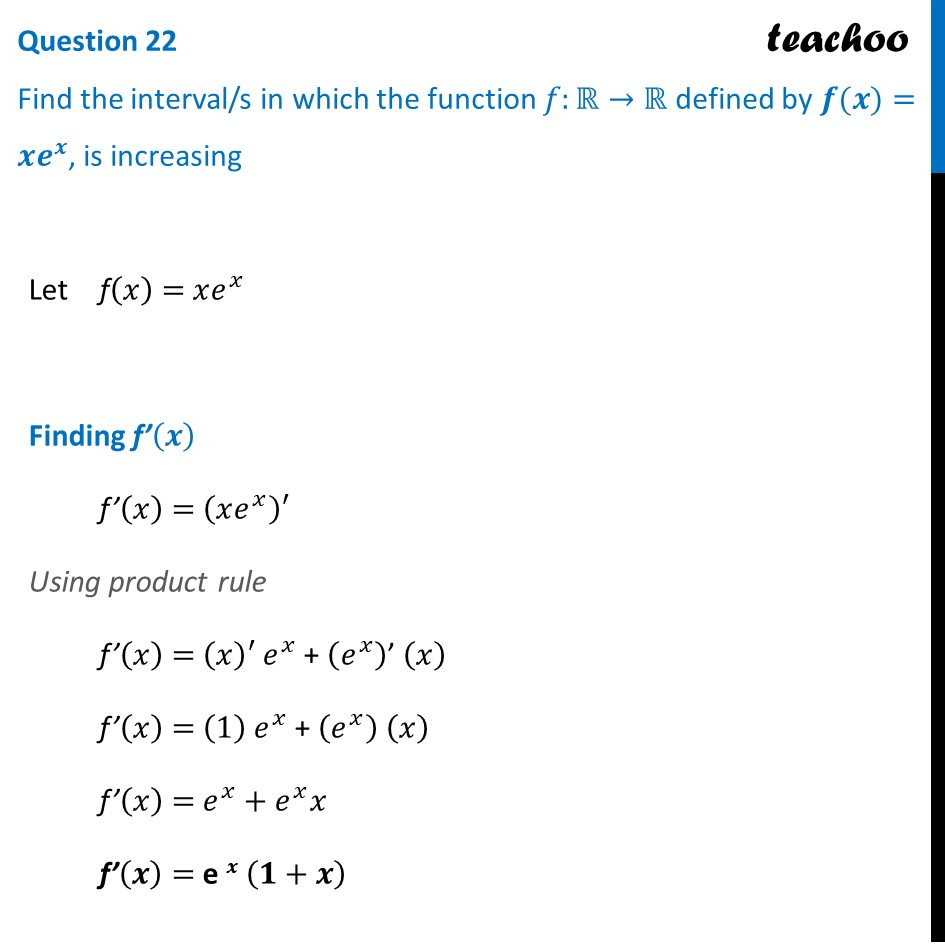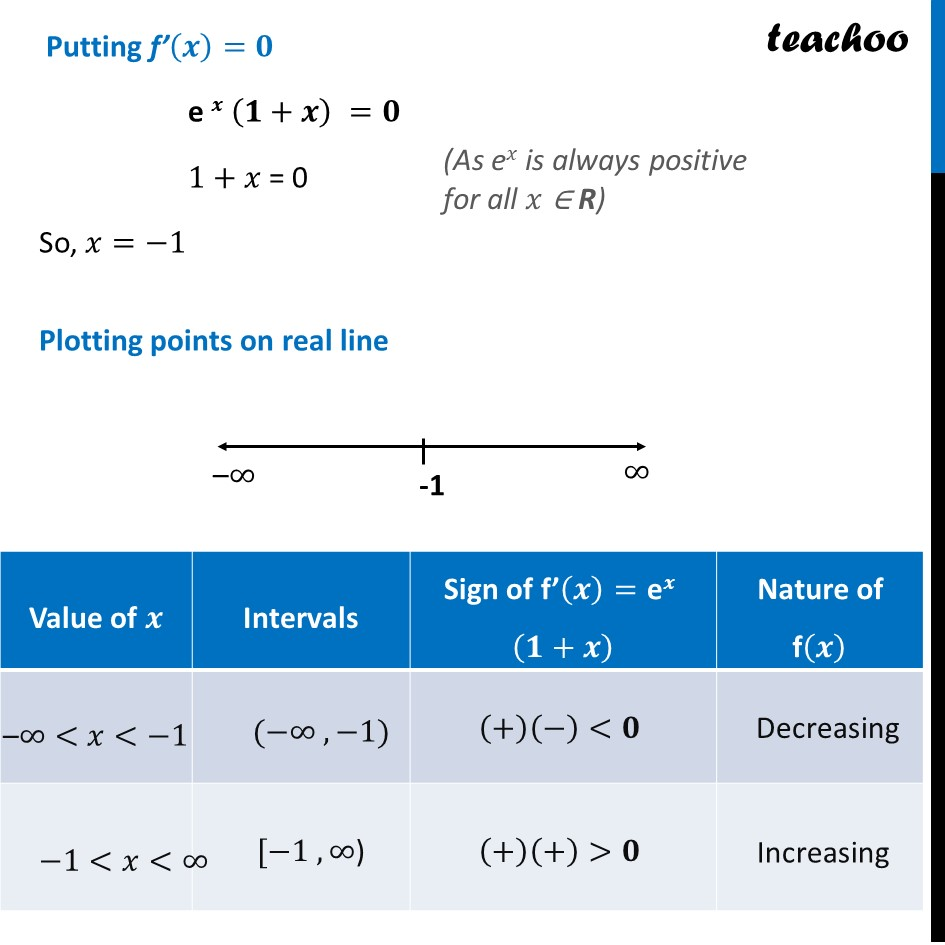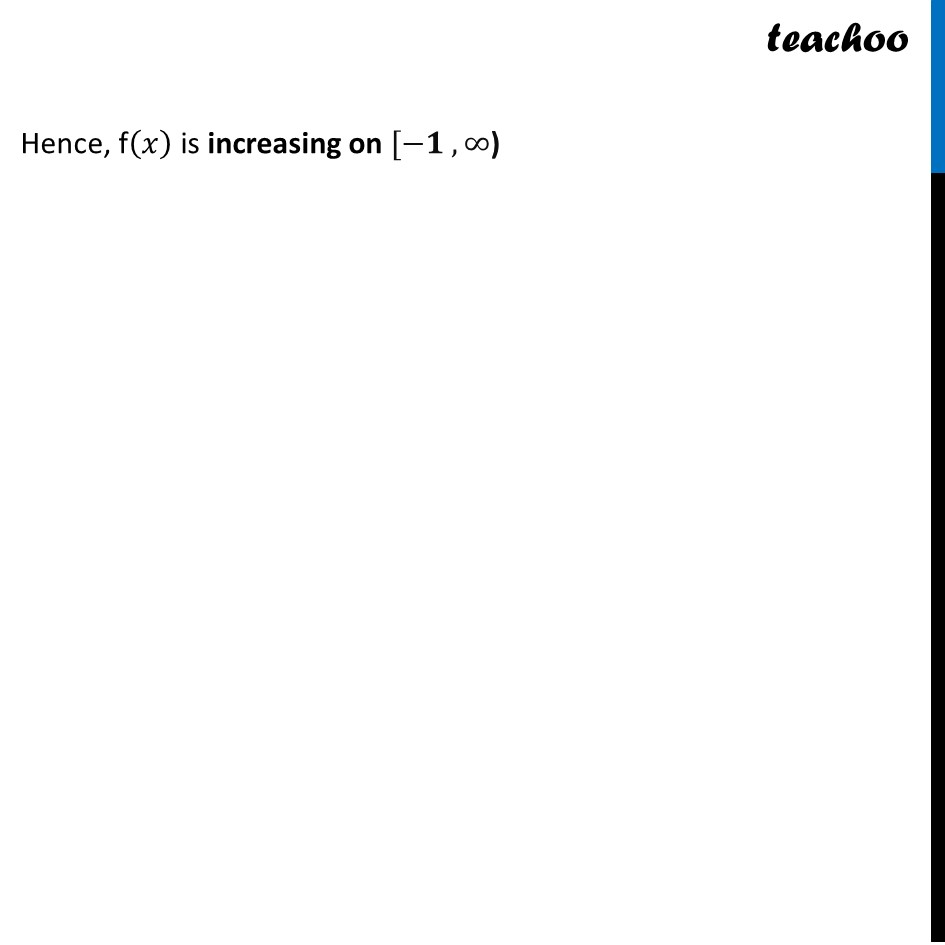CBSE Class 12 Sample Paper for 2024 Boards

Class 12
Solutions of Sample Papers and Past Year Papers - for Class 12 Boards

## Find the interval/s in which the function f:R→R defined by f(x)=xe^x, is increasing

This question is similar to Ex 6.2 ,19 Chapter 6 Class 12Learn in your speed, with individual attention - Teachoo Maths 1-on-1 Class

### Transcript

Let f(𝑥) = 𝑥𝑒^𝑥 Finding f’(𝒙) f’(𝑥) = (𝑥𝑒^𝑥 )′ Using product rule f’(𝑥) = (𝑥)′ 𝑒^𝑥 + (𝑒^𝑥 )’ (𝑥) f’(𝑥) = (1) 𝑒^𝑥 + (𝑒^𝑥 ) (𝑥) f’(𝑥) = 𝑒^𝑥+𝑒^𝑥 𝑥 f’(𝒙) = e 𝒙 (𝟏+𝒙) Putting f’(𝒙)=𝟎 e 𝒙 (𝟏+𝒙) =𝟎 1+𝑥 = 0 So, 𝑥=−1 Plotting points on real line Hence, f(𝑥) is increasing on [−𝟏 ,∞)